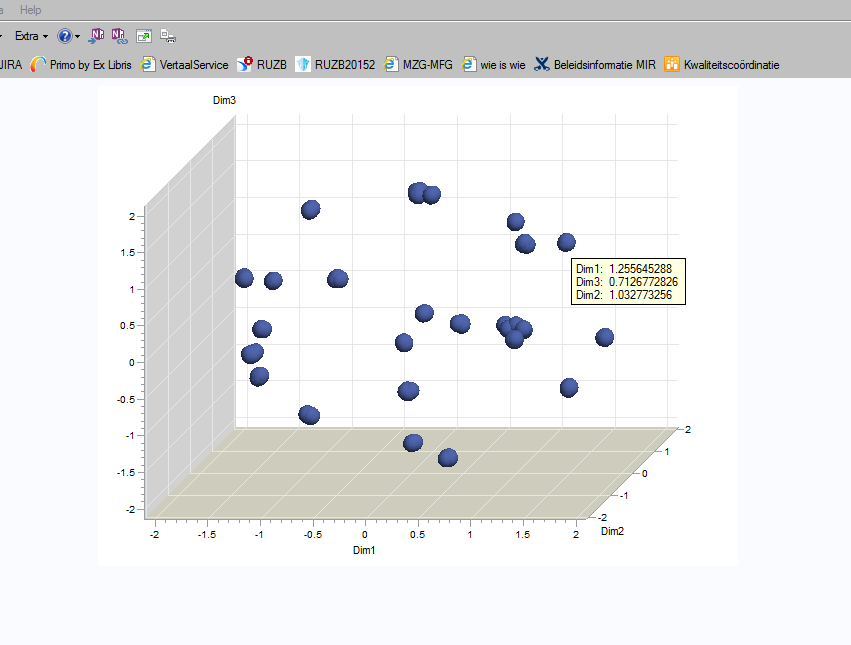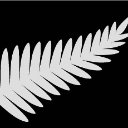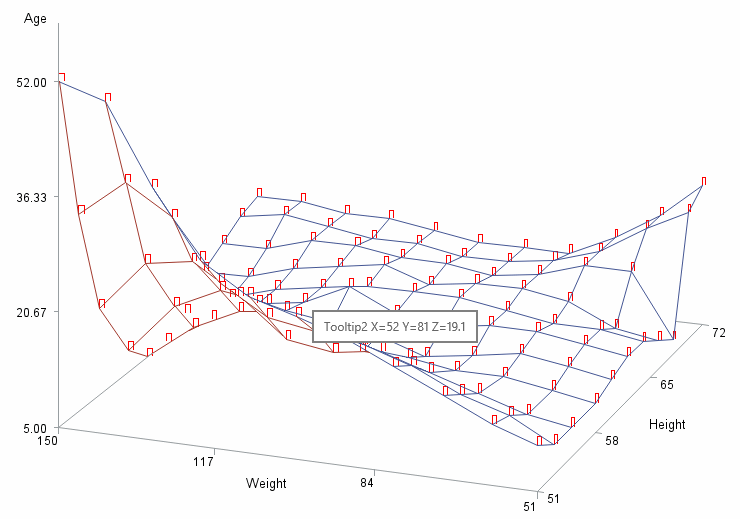## g3d tooltip

hello all,

I would like to visualize in an activx object (in 3d) the clusters of objects (result of proc prinqual and proc fastclus).

in the graph i would like to show as tooltip not the (default) 3 coordinates but some descriptive elements of the object e.g. name, city...

the HTML= options does not work with proc g3d?

this is the code i tried so far...(without and with annote dataset)

data data2;
set data1;
length tips \$ 100;
tips = 'TITLE="Hosp: ' || trim(NameHospital) || '0D'X ||
City: ' || trim(city) || '"';
run;
data anno;
set data2;
x=dim1; y=dim2; z=dim3;
length function \$10 html \$ 100;
function='LABEL';
html = 'TITLE="Hosp: ' || trim(NameHospital) || '0D'X ||
City: ' || trim(city) || '"';
text = html ;
run;

options device = activex;
ods html body = 'C:\temp\SAS_tmp\VZN_threeD.html' ;
ods graphics on / imagemap=on;

proc g3d data = data2 ;
scatter dim2 * dim1 = dim3 / grid noneedle shape="balloon" size=2; * html=tips;
run;
quit;
proc g3d data = data2 ;
scatter dim2 * dim1 = dim3 / ANNO=anno grid noneedle shape="balloon" size=2;
run;
quit;

goptions reset = all;
ods html close;
ods listing;

the tooltips remain unchanged...thanks for any suggestion or advice !

greetings,

Guy

1 ACCEPTED SOLUTION

Accepted SolutionsChrisNZ
Tourmaline | Level 20

## Re: g3d tooltip

The ACTIVEX and ACTXIMG devices do not display custom data tips when the number of observations exceeds 5000.

Are you in this situation?

4 REPLIES 4ChrisNZ
Tourmaline | Level 20

## Re: g3d tooltip

The ACTIVEX and ACTXIMG devices do not display custom data tips when the number of observations exceeds 5000.

Are you in this situation?ChrisNZ
Tourmaline | Level 20

## Re: g3d tooltip

ActiveX doesn't seem too keen on tooltips for 3D plots.

Here is a test using ActiveX and GIF drivers, and using proc GPLOT and proc G3D.

Tooltips show for 3 graphs, but not ActiveX + G3D.

Note that you must draw an area in ANNOTATE for the tooltips to appear. I made the areas visible (red rectangles) in this example, but you can hide them using

``WHEN 'B' COLOR 'white';``

Here is the example:

``````
proc g3grid data= SASHELP.CLASS out=GRID;
grid WEIGHT*HEIGHT=AGE;
run;

data PLOT;
set GRID;
HTML  =catt('title="Tooltip1 Y=',WEIGHT,' X=',HEIGHT,' Z=',AGE,'"');
AGE   =round(AGE);
HEIGHT=round(HEIGHT);
WEIGHT=round(WEIGHT);
run;
data ANNO;
set PLOT;
retain XSYS YSYS ZSYS '2' WHEN 'A' COLOR 'red';
FUNCTION='move'; Y=WEIGHT; X=HEIGHT; Z=AGE;
output;
FUNCTION='bar'; X+1; Y+1; Z+.1; HTML=catt('title="Tooltip2 X=',X,' Y=',Y,' Z=',Z,'"');
output;
run;

symbol1 v=dot h=1;
symbol2 v=dot h=1;
symbol3 v=dot h=1;
symbol4 v=dot h=1;
symbol5 v=dot h=1;

ods html file="%sysfunc(pathname(WORK))\test.html" gpath='.';
goptions dev=activex;
proc gplot data=PLOT; plot WEIGHT*HEIGHT=AGE /html=HTML;run;
proc g3d   data=PLOT; plot WEIGHT*HEIGHT=AGE ;run;

proc gplot data=PLOT anno=ANNO; plot WEIGHT*HEIGHT=AGE ;run;
proc g3d   data=PLOT anno=ANNO; plot WEIGHT*HEIGHT=AGE ;run;

goptions dev=gif;
proc gplot data=PLOT; plot WEIGHT*HEIGHT=AGE /html=HTML name='u1';run;
proc g3d   data=PLOT; plot WEIGHT*HEIGHT=AGE /          name='u2';run;

proc gplot data=PLOT anno=ANNO; plot WEIGHT*HEIGHT=AGE /name='u3';run;
proc g3d   data=PLOT anno=ANNO; plot WEIGHT*HEIGHT=AGE /name='u4';run;
ods html close;``````## Re: g3d tooltip

hi Chris,
thanks, gonna try this !
Guy

## Re: g3d tooltip

hi Chris,

no less than 500

thanks!

Guy

Discussion stats
• 4 replies
• 1109 views
• 0 likes
• 2 in conversation